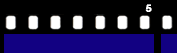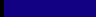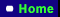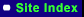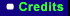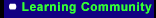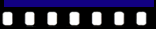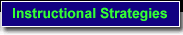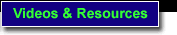Virginia Standards of Learning (SOL K.1) One to One Correspondance (SOL K.1) Comparing More Than, Less Than, Same (SOL 1.2) Grouping Hundreds, Tens, and Ones (SOL 1.3) Skip Counting (SOL 2.1) Identify Place Value to 100's Place (SOL 2.4) Identify and Write Fractions (SOL 3.2) Rounding to Nearest 10 or 100 (SOL 4.9, 5.5) The Division Process: Division with Remainders  SOL K.1 The Student, given two sets containing 10 or fewer concrete items, will identify and describe one set as having more, fewer, or the same number of members as the other set, using the concept of One to One correspondence. Target Skill: One to One Correspondance Math Concept: Place Value, Whole Number Operation SOL K.1 The Student, given two sets containing 10 or fewer concrete items, will identify and describe one set as having more, fewer, or the same number of members as the other set, using the concept of One to One correspondence. Target Skill: Comparing More Than, Less Than, Same Math Concept: Place Value, Whole Number Operation SOL K.2 The student will group concrete objects by ones and tens to develop an understanding of place value. Target Skill: Grouping Hundreds, Tens, and Ones Math Concept: Place Value SOL 1.3 The student will count by twos, fives, and tens to 100. Target Skill:  Skip Counting Math Concept: Whole Number Operation SOL 2.1 The student will identify the place value of each digit in a three-digit numeral, using numeration models. Target Skill: Identify Place Value to 100's Place Math Concept: Place Value SOL 2.4 The student will identify the part of a set and/or region that represents one-half, one-third, one-fourth, one-eighth, and one-tenth and write the corresponding fraction. Target Skill: Identify and Write Fractions Math Concept: Fractions SOL 3.2 The student will read and write six-digit numerals and identify the place value for each digit. Target Skill: Rounding to Nearest 10 or 100 Math Concept: Place Value SOL 3.6 The student will name and write the fractions represented by drawings or concrete materials and symbols. Target Skill: Compare Fractions with Like and Unlike Denominators Math Concept: Fractions SOL 4.2 The student will identify and represent equivalent fractions and relate fractions to decimals, using concrete objects. Target Skill: Identify and Represent Equivalent Fractions Math Concept: Fractions SOL 4.8 The student will find the product of two whole numbers when one factor has two digits or less and the other factor has three digits or less, using estimation and paper and pencil. For larger products (a two-digit numeral times a three-digit numeral), estimation and calculators will be used. Target Skill: Multiplying Two Digit by One Digit Numbers with Regrouping Math Concept: Place Value, Whole Number Operation SOL 4.9, 5.5 The student will estimate and find the quotient of two whole numbers given a one-digit divisor. Target Skill: The Division Process: Division with Remainders  Math Concept: Whole Number Operation SOL 5.7 The student will add and subtract with fractions and mixed numerals, with and without regrouping, and express answers in simplest form. Problems will include like and unlike denominators, limited to 12 or less. Target Skill: Adding and Subtracting Fractions with Mixed Numbers Math Concept: Fractions